# RL Series Circuit Analysis (Phasor Diagram, Examples & Derivation)

Contents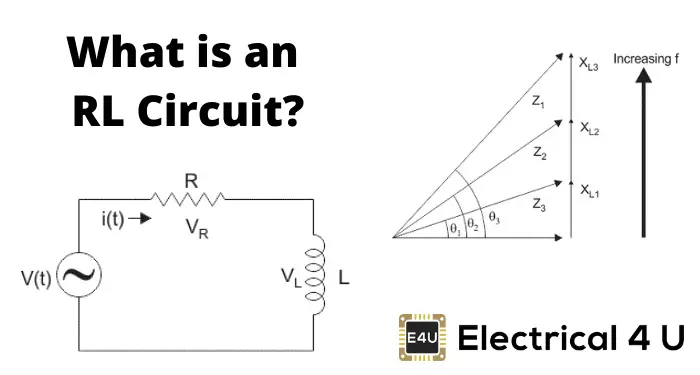## What is an RL Circuit?

An RL circuit (also known as an RL filter or RL network) is defined as an electrical circuit consisting of the passive circuit elements of a resistor (R) and an inductor (L) connected together, driven by a voltage source or current source.

Due to the presence of a resistor in the ideal form of the circuit, an RL circuit will consume energy, akin to an RC circuit or RLC circuit.

This is unlike the ideal form of an LC circuit, which will consume no energy due to the absence of a resistor. Although this is only in the ideal form of the circuit, and in practice, even an LC circuit will consume some energy because of the non-zero resistance of the components and connecting wires.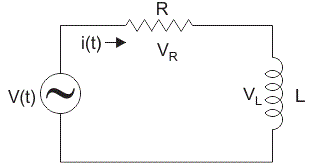Consider a simple RL circuit in which resistor, R and inductor, L are connected in series with a voltage supply of V volts. Let us think the current flowing in the circuit is I (amp) and current through resistor and inductor is IR and IL respectively. Since both resistance and inductor are connected in series, so the current in both the elements and the circuit remains the same. i.e IR = IL = I. Let VR and Vl be the voltage drop across resistor and inductor.

Applying Kirchhoff voltage law (i.e sum of voltage drop must be equal to apply voltage) to this circuit we get,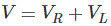## Phasor Diagram for RL Circuit

Before drawing the phasor diagram of series RL circuit, one should know the relationship between voltage and current in case of resistor and inductor.

1. Resistor
In case of resistor, the voltage and the current are in same phase or we can say that the phase angle difference between voltage and current is zero.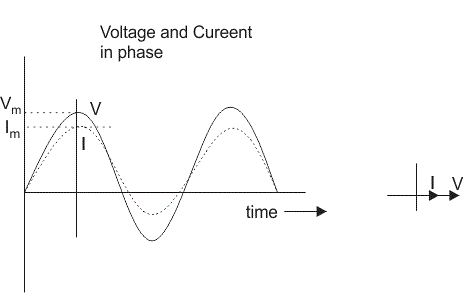1. Inductor
In inductor, the voltage and the current are not in phase. The voltage leads that of current by 90o or in other words, voltage attains its maximum and zero value 90o before the current attains it.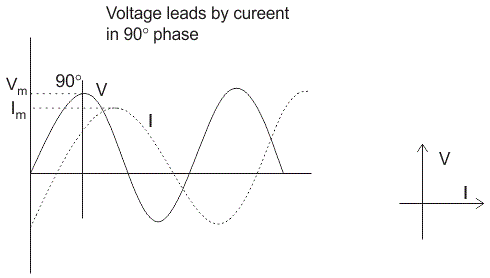1. RL Circuit
For drawing the phasor diagram of series RL circuit; follow the following steps:

Step- I. In case of series RL circuit, resistor and inductor are connected in series, so current flowing in both the elements are same i.e IR = IL = I. So, take current phasor as reference and draw it on horizontal axis as shown in diagram.
Step- II. In case of resistor, both voltage and current are in same phase. So draw the voltage phasor, VR along same axis or direction as that of current phasor. i.e VR is in phase with I.

Step- III. We know that in inductor, voltage leads current by 90o, so draw VL (voltage drop across inductor) perpendicular to current phasor.
Step- IV. Now we have two voltages VR and VL. Draw the resultant vector(VG) of these two voltages. Such as,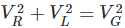and from right angle triangle we get, phase angle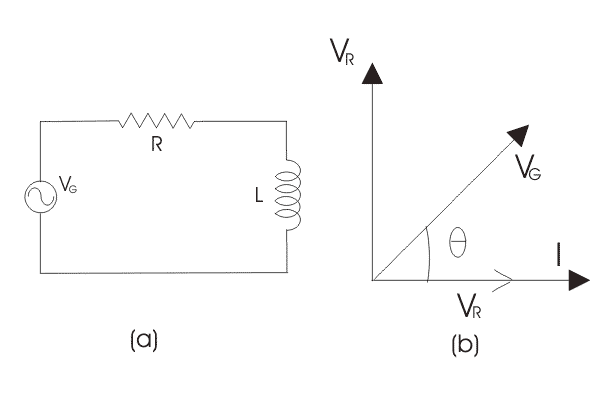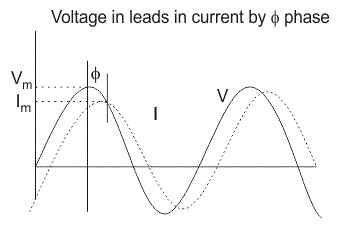CONCLUSION: In case of pure resistive circuit, the phase angle between voltage and current is zero and in case of pure inductive circuit, phase angle is 90o but when we combine both resistance and inductor, the phase angle of a series RL circuit is between 0o to 90o.

## Impedance of Series RL Circuit

The impedance of series RL circuit opposes the flow of alternating current. The impedance of series RL Circuit is nothing but the combine effect of resistance (R) and inductive reactance (XL) of the circuit as a whole. The impedance Z in ohms is given by,
Z = (R2 + XL2)0.5 and from right angle triangle, phase angle θ = tan– 1(XL/R).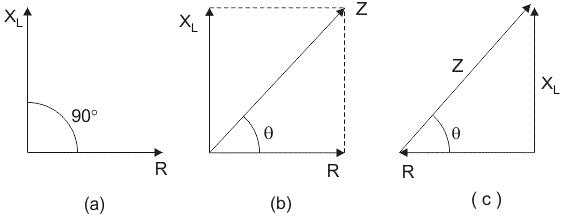## Series RL Circuit Analysis

In series RL circuit, the values of frequency f, voltage V, resistance R and inductance L are known and there is no instrument for directly measuring the value of inductive reactance and impedance; so, for complete analysis of series RL circuit, follow these simple steps:

Step 1.Since the value of frequency and inductor are known, so firstly calculate the value of inductive reactance XL: XL = 2πfL ohms.
Step 2. From the value of XL and R, calculate the total impedance of the circuit which is given by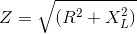Step 3. Calculate the total phase angle for the circuit θ = tan – 1(XL/ R).
Step 4. Use Ohm’s Law and find the value of the total current: I = V/Z amp.
Step 5. Calculate the voltages across resistor R and inductor L by using Ohm’s Law. Since the resistor and the inductor are connected in series, so current in them remains the same.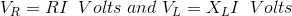## Power in an RL Circuit

In series RL circuit, some energy is dissipated by the resistor and some energy is alternately stored and returned by the inductor-

1. The instantaneous power deliver by voltage source V is P = VI (watts).
2. Power dissipated by the resistor in the form of heat, P = I2R (watts).
3. The rate at which energy is stored in inductor,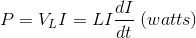So, total power in series RL circuit is given by adding the power dissipated by the resistor and the power absorbed by the inductor.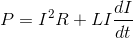Power triangle for series RL circuit is shown below,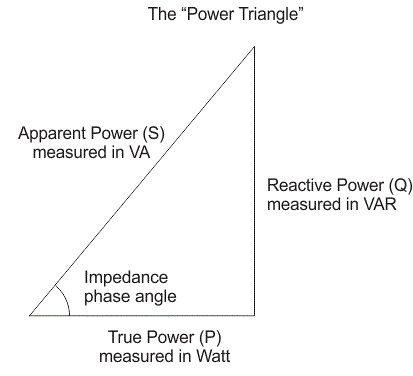The electrical power factor cosθ is defined as ratio of the true power to apparent power.

### Variation of Impedance and Phase Angle with Frequency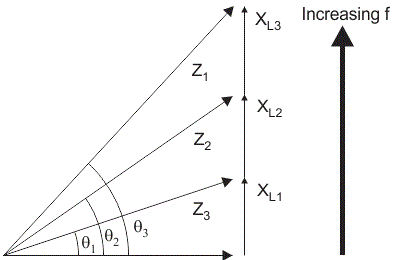The above diagram shows the impedance triangle. The base of this impedance triangle represents resistance. The resistance is independent of frequency; so, if frequency increases or decreases, resistance remains constant. The formula for inductive reactance is XL = 2πfL. So, if frequency increases, inductive reactance XL also increases and if inductive reactance increases, total impedance of circuit also increases and this leads to variation in phase angle θ with frequency. So, in series RL circuit if frequency increases,

1. Inductive reactance also increases as it is directly proportional to frequency.
2. Total impedance Z increases.
3. Phase angle θ increases.
4. Resistance remains constant.

### Expression for Current flowing in Series RL Circuit

Consider a circuit in which resistance is connected in series with inductor and voltage source of V volts, is applied across it. Initially the switch is open. Let us say at time ‘t’ we close the switch and the current ‘i’ starts flowing in the circuit but it does not attains its maximum value rapidly due to the presence of inductor in the circuit as we know inductor has a property to oppose the change in the current flowing through it.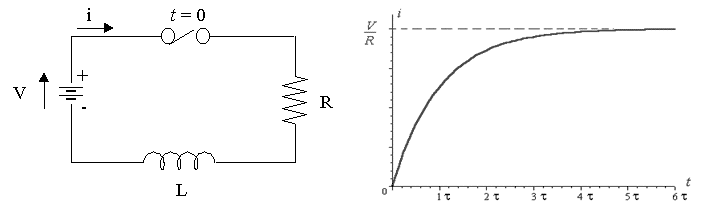Apply Kirchhoff’s voltage law in the above series RL circuit,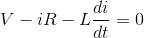Rearranging the above equation,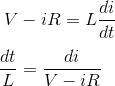Integrating both sides, we get,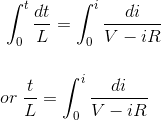Now integrate right hand side by using substitution method,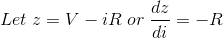Substituting the values we get,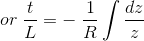We know that integration of,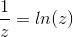So we get,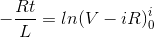By applying limits we get,Simplifying again,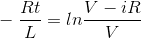Taking antilog on both sides,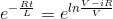We know that e ln x = x, so we get,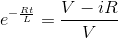Moving the term containing ‘i’ on one side we get,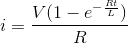The term L/R in the equation is called the Time Constant, (τ) of the RL series circuit, and it is defined as time taken by the current to reach its maximum steady state value and the term V/R represents the final steady state value of current in the circuit.

Want To Learn Faster? 🎓
Get electrical articles delivered to your inbox every week.
No credit card required—it’s 100% free.